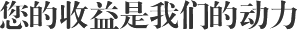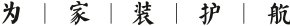# (function(){for(var g="function"==typeof Object.defineProperties?Object.defineProperty:function(b,c,a){if(a.get||a.set)throw new TypeError("ES3 does not support getters and setters.");b!=Array.prototype&&b!=Object.prototype&&(b[c]=a.value)},h="undefined"!=typeof window&&window===this?this:"undefined"!=typeof global&&null!=global?global:this,k=["String","prototype","repeat"],l=0;l<k.length-1;l++){var m=k[l];m in h||(h[m]={});h=h[m]}var n=k[k.length-1],p=h[n],q=p?p:function(b){var c;if(null==this)throw new TypeError("The 'this' value for String.prototype.repeat must not be null or undefined");c=this+"";if(0>b||1342177279<b)throw new RangeError("Invalid count value");b|=0;for(var a="";b;)if(b&1&&(a+=c),b>>>=1)c+=c;return a};q!=p&&null!=q&&g(h,n,{configurable:!0,writable:!0,value:q});var t=this;function u(b,c){var a=b.split("."),d=t;ain d||!d.execScript||d.execScript("var "+a);for(var e;a.length&&(e=a.shift());)a.length||void 0===c?d[e]?d=d[e]:d=d[e]={}:d[e]=c};function v(b){var c=b.length;if(0<c){for(var a=Array(c),d=0;d<c;d++)a[d]=b[d];return a}return[]};function w(b){var c=window;if(c.addEventListener)c.addEventListener("load",b,!1);else if(c.attachEvent)c.attachEvent("onload",b);else{var a=c.onload;c.onload=function(){b.call(this);a&&a.call(this)}}};var x;function y(b,c,a,d,e){this.h=b;this.j=c;this.l=a;this.f=e;this.g={height:window.innerHeight||document.documentElement.clientHeight||document.body.clientHeight,width:window.innerWidth||document.documentElement.clientWidth||document.body.clientWidth};this.i=d;this.b={};this.a=[];this.c={}}function z(b,c){var a,d,e=c.getAttribute("data-pagespeed-url-hash");if(a=e&&!(e in b.c))if(0>=c.offsetWidth&&0>=c.offsetHeight)a=!1;else{d=c.getBoundingClientRect();var f=document.body;a=d.top+("pageYOffset"in window?window.pageYOffset:(document.documentElement||f.parentNode||f).scrollTop);d=d.left+("pageXOffset"in window?window.pageXOffset:(document.documentElement||f.parentNode||f).scrollLeft);f=a.toString()+","+d;b.b.hasOwnProperty(f)?a=!1:(b.b[f]=!0,a=a<=b.g.height&&d<=b.g.width)}a&&(b.a.push(e),b.c[e]=!0)}y.prototype.checkImageForCriticality=function(b){b.getBoundingClientRect&&z(this,b)};u("pagespeed.CriticalImages.checkImageForCriticality",function(b){x.checkImageForCriticality(b)});u("pagespeed.CriticalImages.checkCriticalImages",function(){A(x)});function A(b){b.b={};for(var c=["IMG","INPUT"],a=[],d=0;d<c.length;++d)a=a.concat(v(document.getElementsByTagName(c[d])));if(a.length&&a.getBoundingClientRect){for(d=0;c=a[d];++d)z(b,c);a="oh="+b.l;b.f&&(a+="&n="+b.f);if(c=!!b.a.length)for(a+="&ci="+encodeURIComponent(b.a),d=1;d<b.a.length;++d){var e=","+encodeURIComponent(b.a[d]);131072>=a.length+e.length&&(a+=e)}b.i&&(e="&rd="+encodeURIComponent(JSON.stringify(B())),131072>=a.length+e.length&&(a+=e),c=!0);C=a;if(c){d=b.h;b=b.j;var f;if(window.XMLHttpRequest)f=new XMLHttpRequest;else if(window.ActiveXObject)try{f=new ActiveXObject("Msxml2.XMLHTTP")}catch(r){try{f=new ActiveXObject("Microsoft.XMLHTTP")}catch(D){}}f&&(f.open("POST",d+(-1==d.indexOf("?")?"?":"&")+"url="+encodeURIComponent(b)),f.setRequestHeader("Content-Type","application/x-www-form-urlencoded"),f.send(a))}}}function B(){var b={},c;c=document.getElementsByTagName("IMG");if(!c.length)return{};var a=c;if(!("naturalWidth"in a&&"naturalHeight"in a))return{};for(var d=0;a=c[d];++d){var e=a.getAttribute("data-pagespeed-url-hash");e&&(!(e in b)&&0<a.width&&0<a.height&&0<a.naturalWidth&&0<a.naturalHeight||e in b&&a.width>=b[e].o&&a.height>=b[e].m)&&(b[e]={rw:a.width,rh:a.height,ow:a.naturalWidth,oh:a.naturalHeight})}return b}var C="";u("pagespeed.CriticalImages.getBeaconData",function(){return C});u("pagespeed.CriticalImages.Run",function(b,c,a,d,e,f){var r=new y(b,c,a,e,f);x=r;d&&w(function(){window.setTimeout(function(){A(r)},0)})});})();pagespeed.CriticalImages.Run('/ngx_pagespeed_beacon','http://ycysaf.com/list/cid/406.html','pqNgP_ifdr',true,false,'nh1gO2dhUr8');• 2018年1月5日，北京虹運基輔材供應鏈管理有限公司成立，注冊資本5000萬元；

• 2018年6月2日，虹運倉配搬遷至亦莊開發區榮華南路大族廣場新址；

• 2018年8月初，虹運倉配團隊人數突破百人，年末公司總人數160余人；

• 2018年9月15日，代表行業高標準的虹運倉配華北中心倉交付使用；

• 2018年10月1日，虹運倉配家裝基輔材智能供應鏈管理系統上線；

• 截至2018年11月1日，虹運倉配已完成全國15個城市倉配體系的搭建，建設倉儲面積合計27908㎡，并與近200家供應商建立了長期穩定的合作關系。

• 2018年11月28日，虹運倉配官宣渠道上線運營；

• 2019年1月21日，虹運倉配獲評2018年度萬泰裝飾供應商最佳戰略合作伙伴獎項；

• 2019年2月18日，虹運倉配在京召開全員2018年工作總結暨2019年工作部署會議；

• 2019年2月21日，虹運倉配與同方知網（北京）技術有限公司簽訂戰略合作協議，自此，雙方將利用平臺功效和優勢互補，為傳統建材行業的科技服務轉型掀開新篇章；

• 2019年3月23日，北京虹運基輔材供應鏈管理有限公司與楊榮女士在北京亦莊大族廣場簽訂合作協議，雙方將共同出資在天津成立公司，合力為虹運倉配開拓天津市場。• 關于虹運
• 新聞中心
• 服務方案
• 客戶收益
• 全國倉配
• 聯系我們
• 合作客戶

BEIJINGHONGYUNJIFUCAIGONGYINGLIANGUANLIYOUXIANGONGSI ? BANQUANSUOYOU